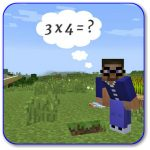Home /This course requires an iPad for the Splash Math Worksheets, in addition to computer Minecraft.

Each week your student will learn one or more new concepts and then practice what they have learned in Splash Math.  This is a full 30 week math program that you purchase in 6 week increments.

You may start in any session. Once the math concepts are mastered, your student will apply what they have learned in the Minecraft world.

PLEASE NOTE: All math courses require an iPad and the purchase of Splash Math from the app store for \$9.95.  Please do not let your student start the app prior to the class.

Splash math is a fun and interactive “smart learning system” that allows students to earn stars to unlock special prizes and games. Each star takes approximately 10 to 20 minutes of gameplay to earn.  The numbers in parentheses below indicate the  number of stars your student will learn in each topics for the given week.  Find out more by clicking here to visit the Splash Math website.

### Unit 1

Week 1 – Place Value (3), Algebra (6)
Week 2 – Place Value (3), Addition (6)
Week 3 – Number Sense (3), Subtraction (6)
Week 4 – Number Sense (3), Algebra (6), Geometry (3)
Week 5 – Number Sense (3), Multiplication (6)
Week 6 – Capstone Project

### Unit 2

Week 1 – Algebra (3), Multiplication (3), Decimals (3)
Week 2 – Multiplication (3),  Fractions (6), Decimals (3)
Week 3 – Division (3), Fractions (3), Measurements (3)
Week 4 – Multiplication (3), Decimals (6)
Week 5 – Division (3), Fractions (6)
Week 6 – Capstone Project

### Unit 3

Week 1 – Multiplication (3), Decimals (6), Geometry (3)
Week 2 – Division (3), Fractions (6)
Week 3 – Multiplication (3), Decimals (6)
Week 4 – Division (3), Measurements (6)
Week 5 – Multiplication (3), Decimals (6), Geometry (3)
Week 6 – Capstone Project

### Unit 4

Week 1 – Multiplication (3), Decimals (3), Measurements (3)
Week 2 – Division (3), Fractions (3), Fraction Arithmetic (3)
Week 3 – Multiplication (3), Fraction Arithmetic (3), Measurements (3)
Week 4 – Division (3), Fractions (3), Geometry (3), Geometry (3)
Week 5 – Multiplication (3), Measurements (6)
Week 6 – Capstone Project

### Unit 5

Week 1 – Multiplication (6), Fraction Arithmetic (3)
Week 2 – Division (3) Fraction Arithmetic (6)
Week 3 – Multiplication (3), Fraction Arithmetic (3), Measurements (3)
Week 4 – Fraction Arithmetic (6), Geometry (6)
Week 5 – Multiplication (3), Fraction Arithmetic (3), Measurements (3)
Week 6 – Capstone Project

Course Information

• Course Code: Splash-4th
• Course Location: Online Course + Minecraft
• Course Delivery: Assignment Portal
• Course Duration: 6 weeks